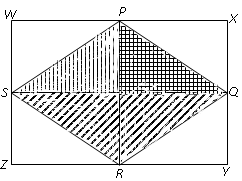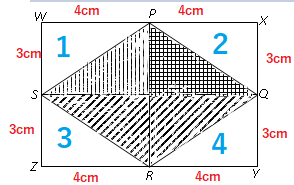mycollegehive
Calculate the area of the part of the rectangle that is not shaded
waec-2018 ssce-exam-2018 waec-mathematics-2018 math0In the diagram WXYZ is a rectangle with dimension 8 cm by 6 cm. P, Q,  R and S are the midpoint s of the sides of the rectangle as shown.

Calculate the area of the part of the rectangle that is not shaded

A:  25 cm2

B:  24 cm2

C:  16 cm2

D:  12cm2

67 viewsShareFollowUniversity of Lagos Nigeria
15 July 2020University of Benin Nigeria
15 July 20200Line WX = ZY = 8cm

Line WZ = XY = 6cm

Since Line SQ cuts across the middle of line WZ and XY, Line WS = SZ = XQ = QY = 3cm

Also, Line PR cuts across the middle of Line WX and ZY, thus, Line WP = PX = ZR = RY = 4cm.

There are 4 section of the un-shaded region with each forming a right angle triangle of same dimensions.Therefore, the area of the un-shaded portions will simply be 4 times the area of a triangle. That is:

4(1/2 x base x height)

= 4(1/2 x 4 x 3)

= 4 (6)

= 24cm2

Ans BShare### Related Tags

waec-2018

0 followers

29 questionsssce-exam-2018

0 followers

29 questionswaec-mathematics-2018

0 followers

28 questions